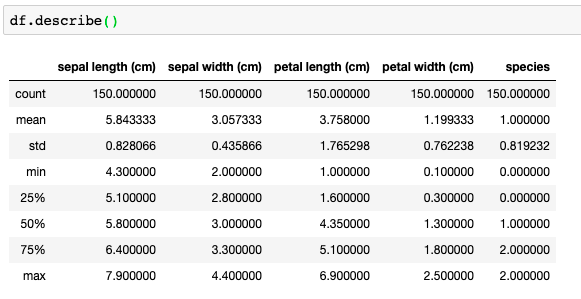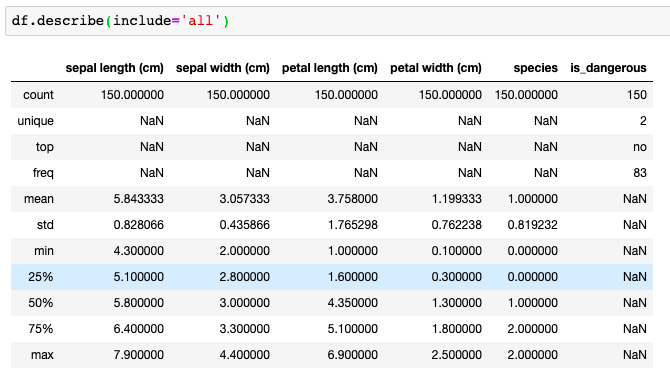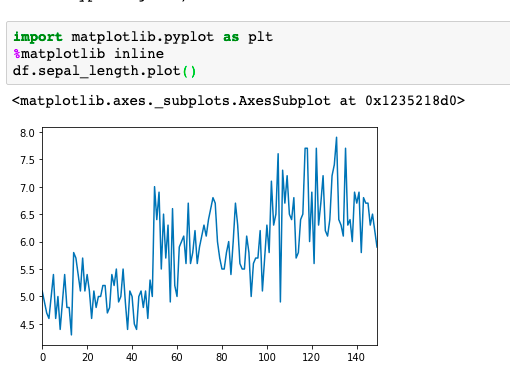Search
•Magdalena Konkiewicz

# 7 practical pandas tips when you start working with the libraryIntroduction

In this article, I would like to share some tips that I think are useful for anyone that starts their journey with Data Science. Python pandas package is one of the most used libraries by Data Scientists and is definitely on the must list for anyone who wants to be involved in the field.

Sometimes the learning journey is not so straight forward so I would like to share some tips that will make it easier. Some of the tips are more traditional usages of some of the pandas most important functions when the other ones are practical advice on how to do some things within the package.

Use this 3 functions to examine your data: head(), info(), describe()

These are three functions that will help you with initial data analysis and already will give you a lot of information about the data set. It is always a good start after loading the data set to run all three.

We are going to load the iris data set to demonstrate how they work. You would probably do this just after loading your csv file.

```
from sklearn.datasets import load_iris
import pandas as pd
df = pd.DataFrame(data['data'], columns=data['feature_names'])
df['species'] = data['target']

```

Now that the data is loaded you can see how the head function works:You can see that it shows the first 5 rows of the data frame together with its column names on top and indexes on the left side. This is some interesting information already.

Let’s now try to use info() function:This gives us information about the number of rows, 150 entries, and the number of columns which is 5. You can see what data types are in our columns. In this example, all columns are floats except the species column which is an integer.

Let’s see what describe() function can add here:There is much more information here. We can see what are the maximum and minimum value for each column. We can also read central tendency summary: mean and standard deviation, and also interquartile ranges (25%, 50. 75%).

There is one more thing that is not explored here. In my data set, all the columns are numerical and describe() function works differently for numerical and categorical variables. To demonstrate how to use both I will add a fake categorical column called ‘is_dangerous’.

```
import numpy as np
df['is_dangerous'] = np.where(df['sepal length (cm)']>6, 'yes', 'no')```

I have just made all rows that have a sepal length greater than 6 dangerous. Let’s call describe now.Notice I have used include parameter with describe() and I have set it to ‘all’. This makes the function to include data description for categorical variables as well. You can see that is_dangerous column has 2 unique values, the most common is ‘no’ and it occurs 83 times.

I hope you can see that just with these three functions: head(), info(), and describe() you can learn a lot about the data.

How to see all columns in a data frame

If you have many columns in your data frame the default behavior in jupyter notebook is to show you some first and last columns and three dots in the middle (…). The same is true if your data frame has many rows and you want to see all of them. The easy fix is to check the dimensions of your data frame and change the default display settings using the following code:

```
pd.set_option('display.max_columns', 500)
pd.set_option('display.max_rows', 500)

```

Add this just after your imports in the code and set the numbers to your data frame dimensions.

Rename your column names if necessary

If your column names are lengthy, include spaces, or just are not good column names the best way is to rename them. Doing this at the beginning of your analysis will solve a lot of problems. Let’s look at default column names in the iris data set.

You can see that column names here are not ideal. I think the metric (cm) is redundant and I would recommend using underscores instead of spaces. This will allow you to select a column in the data frame using a dot notation later on. Let’s change the names then:

```
df.columns = df.columns.str.replace(' \(cm\)', '').str.replace(' ', '_')

```

I have removed ‘ (cm)’ from column names and replaced empty spaces with underscores. The common practice would be removing trailing spaces from column names if there were present. Also if the column names do not follow a pattern that you can apply to all of them you can change them just one by one.

Use built-in graphing functionality for simple data graphs

To do simple graphing you can use just use the build-in pandas functions. Pandas functions are somehow limited and for further analysis, you may need to learn how to use matplotlib or seaborn but if you want to make a quick graph to have a look at your data you can use these one-liners. Let’s explore them with the Iris data set again. I am going to plot the sepal length of all examples in the data set:The x-axis here represents indexes (individual examples) and the y-axis sepal length. Remember to add ‘%matplotlib inline’ after matplotlib import so your graphs are shown within the notebook.

You can also scatter the two variables against each other. Let’s show this by scattering sepal length against sepal width.Another useful pandas graph is a histogram and it can be applied to the whole data frame. That way it will draw histograms for all numeric columns:For visualizing categorical data you can use value_counts() function and bar chart graph from pandas:You can see that you can definitely do some simple graphs with pandas library. Dig in further into the documentation to explore more and learn how to use different parameters to make your graphs even better.

Understand how loc and iloc selection works and be able to use it confidently

Loc and iloc are ways of selecting data from the data frame. The loc one allows you to access data by names (column names and index names) whereas iloc does the same but you need to use integer indexes for both columns and indexes (hence name iloc indicating int number).

Let’s see how they work in practice:

```
df.loc[:, ['sepal_length', 'sepal_width']]

```

The code above selects all rows (colon sign is a shortcut for all) for two columns sepal_length and sepal_width.

```
df.loc[10:50, ['sepal_length', 'sepal_width']]

```

The code above selects the same columns but only rows between 10 and 50. It may be confusing that we use integers for selecting rows here while we use loc. This is because our indexes are integers as we have used default indexing when creating a data frame. You could have indexes that are strings e.g unique row identifier. To showcase this let’s change our indexes to be strings:I have changed indexes to strings now and called the head function to see some data entries.

At first glance, the data frame looks the same but I want to show that there is an important difference. Let’s try the same selection as in the previous example:You should be getting errors when trying this code. I am not going to dive in detail of the error but the reason for this is the fact that with the loc you need to use the specific index values and those are strings now, not integers. However, you can use iloc to do the same operation.Can you see that we had to change the column names to corresponding column numbers? This is because iloc will not work with column names but their indexes (first columns starting at 0).

You can find more detailed tutorials on data frame selections on Towards Data Science but the main point I want to make is that you want to understand how to use them as quickly as possible so you can manipulate your data frames quickly and efficiently.

axis: 0 for rows and 1 for columns

This is rather a quick practical tip for people who do not remember. In pandas always the first thing we refer to is the row and then the column. So if df.shape gives as (160, 2) it means there are 160 rows and 2 columns. When we use the loc and iloc the first thing in square brackets refer to rows and second to columns. When we use pandas function that requires axis parameters such as apply() then axis=0 means rows and axis=1 means columns.

Use autocompletion

My last tip is to use autocompletion within jupyter notebook. It is built-in and you just need to hit the tab button when you start typing your variable to activate it. It will save you time typing and debugging typos. If you still do not know how to use it check the jupyter autocompletion article I have written a while ago.

Conclusion

I have given you some practical tips on how to use pandas and now it is time to try them on some real data set. It is definitely not an exclusive list and there is still a lot more to learn and cover. I thought this could put you on the right track and clear out some initial confusion when staring with a new library.

Good luck!